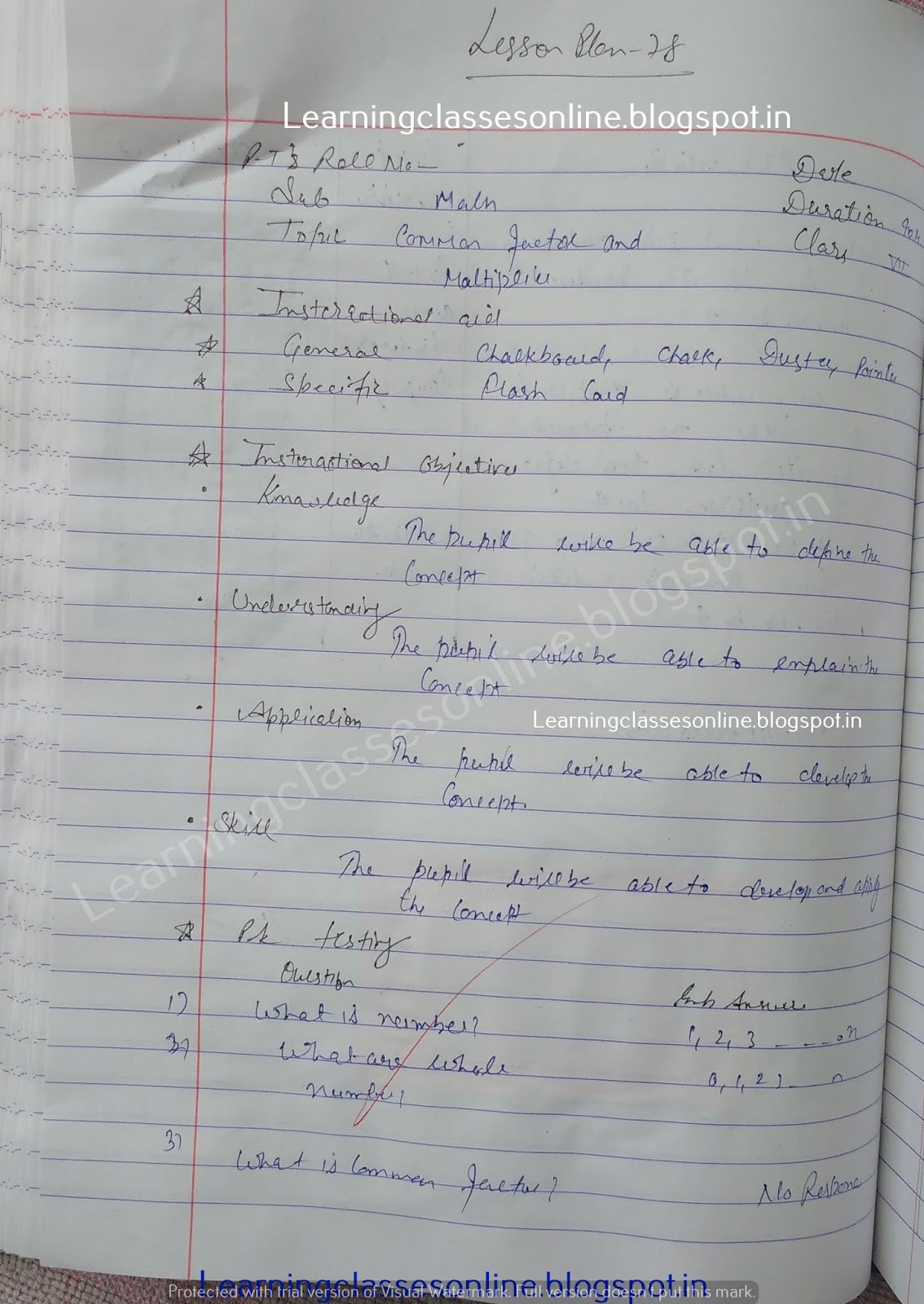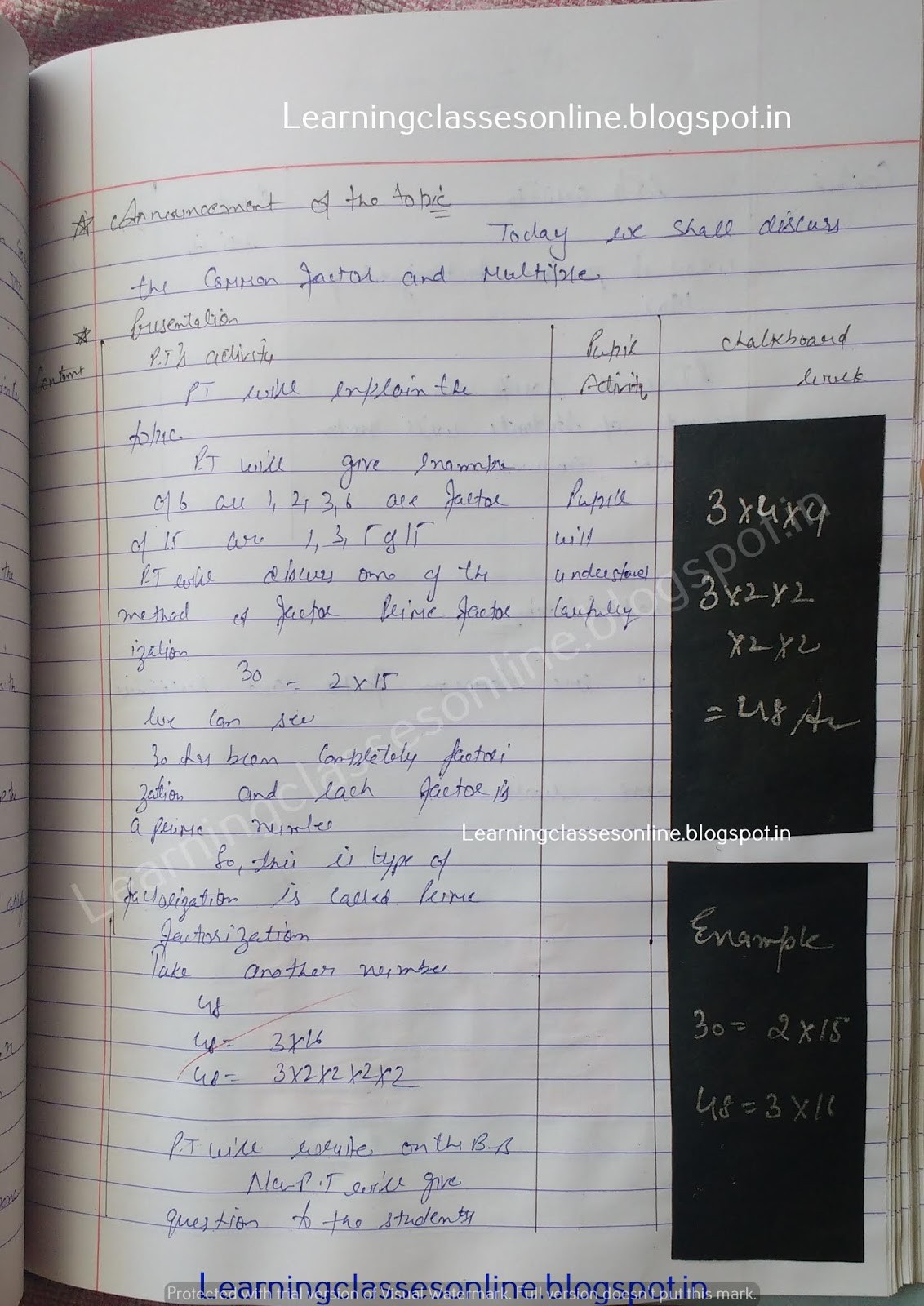Maths Lesson Plan on Common factor and multiply

# Maths Lesson Plan on Common factor and multiply

Maths Lesson Plan on Common factor and multiply for middle and high school mathematics teachers.

Note: The Mathematics Lesson Plan given below is just an example. You can change the Name, Class, Course, Date, Duration, etc. according to your needs.